## Math

### Math Kindergarten

Placement Information: Saxon books are skill-level books, not grade-level books. It is essential that each student is placed in the text that meets the skill level of the individual.Required Textbook: Kindergarten-1st Grade Saxon Math Course -Saxon Math: Level One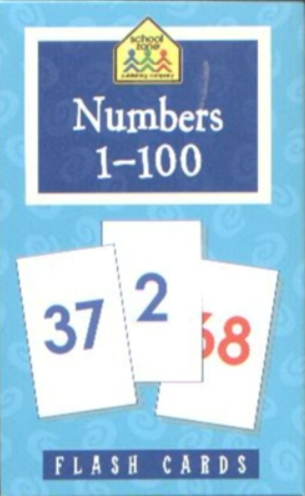Optional:

This level teaches children to skip count by one’s, two’s, five’s and tens, identify ordinal position to tenth, master all basic addition and subtraction facts, measure using inches, feet, and centimeters. Also teaches how to add quarters, draw polygons, and observe information from real graphs, pictographs, and bar graphs.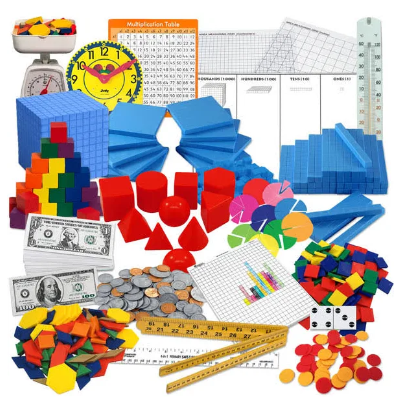Students will develop skills including oral counting; recognizing and sequencing numbers; identifying ordinal position; acting out addition and subtraction stories; counting with one-to-one correspondence; sorting; patterning; graphing real objects and pictures; identifying and counting pennies, nickels, and dimes; identifying one half; identifying shapes; covering and replicating geometric designs; measuring using nonstandard units of measure; telling time to the hour; and using a calendar. Oral assessments are built into the program.

This is a live class that will meet weekly for 30 weeks, beginning the first week of September (see the posted Academic Schedule for holidays). Classes will be held weekly on the same day and time each week for a one-hour live class. We will be using Saxon Math materials (Kit Level one), Number Flashcards (1-100), and the Saxon Math Manipulative kit.

Placement Information: Saxon books are skill-level books, not grade-level books. It is essential that each student is placed in the text that meets the skill level of the individual.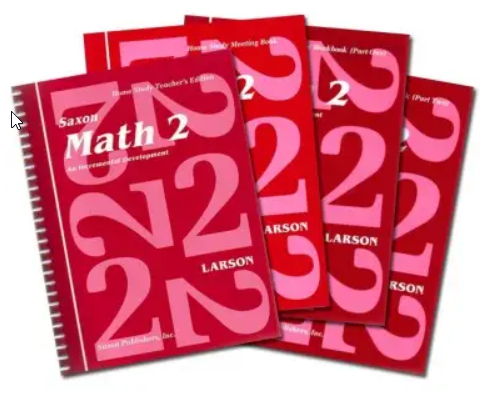Required Textbook:  Saxon Math Level 2

Optional:

Saxon Math Manipulative Kit for Pre-K to 2nd Grademanipulatives are an additional purchase, however, highly recommended.Students will skip count by 1’s, 2’s, 3’s, 4’s, 5’s, 10’s, 25’s, and 100’s; compare and order numbers; identify ordinal position to tenth; identify sorting and patterning rules; solve routine and nonroutine problems; master all basic addition and subtraction facts; master multiplication facts to 5; add and subtract two-digit numbers; picture and name fractions; measure to the nearest centimeter, foot, and half inch; compare volume; compare and measure mass; measure perimeter and area; tell time to five-minute intervals; count pennies, nickels, dimes, and quarters; identify geometric solids; identify lines of symmetry; identify angles; tally; and create, read, and write observations from real graphs, pictographs, bar graphs, Venn diagrams, and line graphs.

This is a live class that will meet weekly for 30 weeks, beginning the first week of September (see the posted Academic Schedule for holidays). Classes will be held weekly on the same day and time each week for a one-hour live class. We will be using Saxon math (Kit Level 2) and the Saxon Math Manipulative Kit.

Placement Information: Saxon books are skill-level books, not grade-level books. It is essential that each student is placed in the text that meets the skill level of the individual.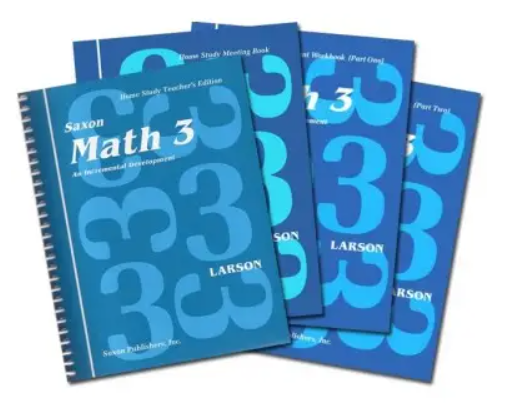Required Textbook: Saxon Math Level 3

Optional:Students will use simulations and games to learn and practice new mathematical concepts. Social studies and science connections to mathematics are stressed. Children will compare and order numbers; identify place value; identify ordinal position to twentieth; identify and complete patterns; solve routine and nonroutine problems; master all basic addition, subtraction, multiplication, and division facts; add and subtract multidigit numbers; multiply a multidigit number by a single-digit number; divide by single-digit divisors; add positive and negative numbers; picture, name, and order fractions; add and subtract fractions with common denominators; measure to the nearest quarter inch, millimeter, foot, and yard; identify volume of standard containers; compare and measure mass; measure perimeter and area; tell time to the minute; determine elapsed time; count money; make change for a dollar; identify angles; identify lines of symmetry; identify function rules; graph ordered pairs on a coordinate graph; tally; and create, read, and write observations from real graphs, pictographs, bar graphs, Venn diagrams, and line graphs.

Live classes will meet weekly, 30 weeks, beginning the first week of September (see the posted Academic Schedule for holidays) on the same day and time each week, for a one-hour-live class. At that class the tutor will: explain the assignment for the coming week; answer your questions and perhaps pose some; note and address any problems or difficulties noticed in the prior week’s work; and lead a brief discussion of the topic at hand. These are rather informal and friendly live tutoring sessions.

Placement Information: Saxon books are skill-level books, not grade-level books. It is essential that each student is placed in the text that meets the skill level of the individual.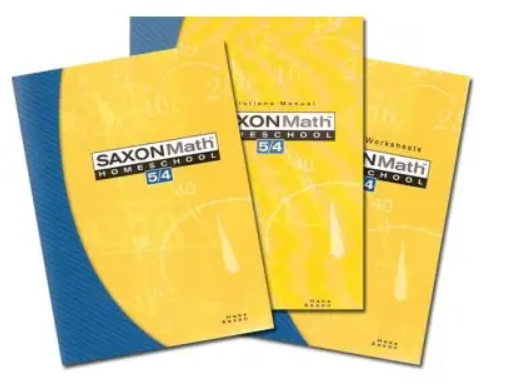Required Textbook: Saxon Math Level 5/4

Math 5/4 is made up of five instructional components: Facts Practice, Mental Math, and Problem Solving; Daily Lessons; Daily Practice; Daily Problem Sets; and Cumulative Tests.

Math 5/4 contains a thorough review of concepts and procedures related to whole-number operations, including single-digit multiplication and division. Word problems are incrementally developed and continually practiced throughout the year. The textbook contains no answers, but an answer key is provided for all homework problems. Math 54 is a balanced, integrated mathematics program that includes incremental development of:

• whole-number concepts and computation
• mental math problem solving
• patterns and functions
• measurement
• geometry
• fractions
• decimals
• statistics and probability

Math is a building skill. Please note it is a crucial to complete all the lessons and investigations. If the student skips or goes out of sequence they may meet problems; all lessons must be completed by students to build on skills, then the student is equipped to solve. On occasion some lessons involve activities with the use of household materials.

Children will skip count by whole numbers; compare and order numbers; identify place value; identify ordinal position to twentieth; identify and complete patterns; solve routine and nonroutine problems; master all basic addition, subtraction, multiplication, and division facts; add and subtract multidigit numbers; multiply a multidigit number by a single-digit number; divide by single-digit divisors; add positive and negative numbers; picture, name, and order fractions; add and subtract fractions with common denominators; measure to the nearest quarter inch, millimeter, foot, and yard; identify volume of standard containers; compare and measure mass; measure perimeter and area; tell time to the minute; determine elapsed time; count money; make change for a dollar; identify angles; identify lines of symmetry; identify function rules; graph ordered pairs on a coordinate graph; tally; and create, read, and write observations from real graphs, pictographs, bar graphs, Venn diagrams, and line graphs.  Children practice skills relating to time, temperature, money, counting, patterning, and problem solving.

A complete set of all necessary student materials is bound in two consumable workbooks. Student materials include written practice pages, masters, and fact cards for Number Fact Practice.

Live classes will meet weekly, 30 weeks, beginning the first week of September (see the posted Academic Schedule for holidays) on the same day and time each week, for a one-hour-live class. At that class, the tutor will: explain the assignment for the coming week; answer your questions and perhaps pose some; note and address any problems or difficulties noticed in the prior week’s work; and lead a brief discussion of the topic at hand. These are rather informal and friendly live tutoring sessions.

Placement Information: Saxon books are skill-level books, not grade-level books. It is essential that each student is placed in the text that meets the skill level of the individual.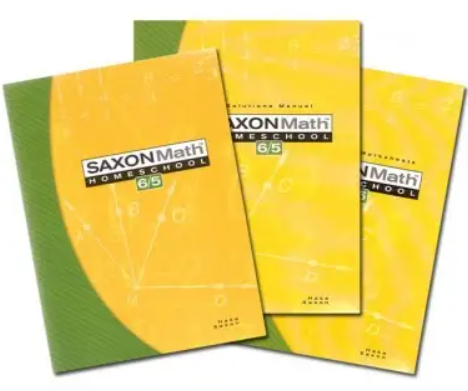Required Textbook: Saxon Math 6/5

Math 6/5 is made up of five instructional components: Facts Practice, Mental Math, and Problem Solving; Daily Lessons; Daily Practice; Daily Problem Sets; and Cumulative Tests.

Math 6/5 is an integrated basic mathematics course that reviews and expands upon all the mathematical content from Math 5/4. The emphasis on problem solving continues as students are called upon to apply mathematical tools and techniques to real-life mathematical situations expressed through word problems.

Math 6/5 includes incremental development of:

• whole-number concepts and computation
• mental math patterns and functions
• measurement
• statistics and probability
• fractions
• mixed numbers
• decimals
• geometry
• percents
• negative numbers
Live classes will meet weekly, 30 weeks, beginning the first week of September (see the posted Academic Schedule for holidays) on the same day and time each week, for a one-hour-live class. At that class, the tutor will: explain the assignment for the coming week; answer your questions and perhaps pose some; note and address any problems or difficulties noticed in the prior week’s work; and lead a brief discussion of the topic at hand. These are rather informal and friendly live tutoring sessions.

Placement Information: Saxon books are skill-level books, not grade-level books. It is essential that each student is placed in the text that meets the skill level of the individual.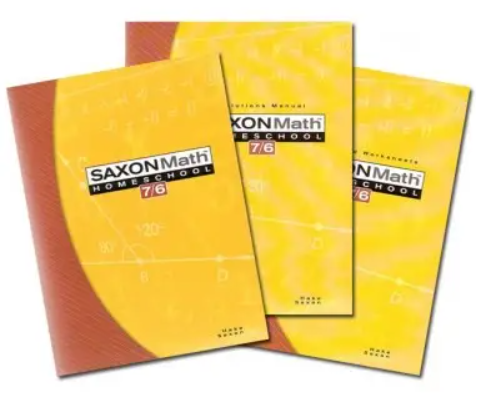Required Textbook: Saxon Math 7/6

Saxon Math 7/6 will help middle-school students gain a solid foundation of algebraic reasoning and geometric skills as they prepare to move on to higher-level math and algebraic reasoning. Covering  fractions, decimals, percents, geometry, area, volume, ratio, proportion, exponents, scientific notation, signed numbers, algebraic terms, 2-step equations and inequalities, slope, graphing, the Pythagorean Theorem, functions and coordinate graphing, integers, exponential expressions, and prime factorization. Students will specifically learn about the order of operations, number lines, decimal place value, how to find the percent of a number, how to round decimal numbers, attributes of geometric solids, and more.

Live classes will meet weekly, 30 weeks, beginning the first week of September (see the posted Academic Schedule for holidays) on the same day and time each week, for a one-hour-live class. At that class, the tutor will: explain the assignment for the coming week; answer your questions and perhaps pose some; note and address any problems or difficulties noticed in the prior week’s work; and lead a brief discussion of the topic at hand. These are rather informal and friendly live tutoring sessions.

Placement Information: Saxon books are skill-level books, not grade-level books. It is essential that each student is placed in the text that meets the skill level of the individual.Required Textbook: Saxon Math 8/7

Saxon Math 8/7 teaches math with a spiral approach, which emphasizes incremental development of new material and continuous review of previously taught concepts. Building upon the principles taught in Saxon Math 7/6, the Saxon 87 textbook reviews arithmetic calculation, measurements, geometry and other skills, and introduces pre-algebra, ratios, probability and statistics. Students will specifically learn about adding/subtracting/multiplying fractions, equivalent fractions, the metric system, repeating decimals, scientific notation, Pi, graphing inequalities, multiplying algebraic terms, the Pythagorean Theorem, the slope-intercept form of linear equations, and more.

Live classes will meet weekly, 30 weeks, beginning the first week of September (see the posted Academic Schedule for holidays) on the same day and time each week, for a one-hour-live class. At that class the tutor will: explain the assignment for the coming week; answer your questions and perhaps pose some; note and address any problems or difficulties noticed in the prior week’s work; and lead a brief discussion of the topic at hand. These are rather informal and friendly live tutoring sessions.

Placement Information: Saxon books are skill-level books, not grade-level books. It is essential that each student is placed in the text that meets the skill level of the individual.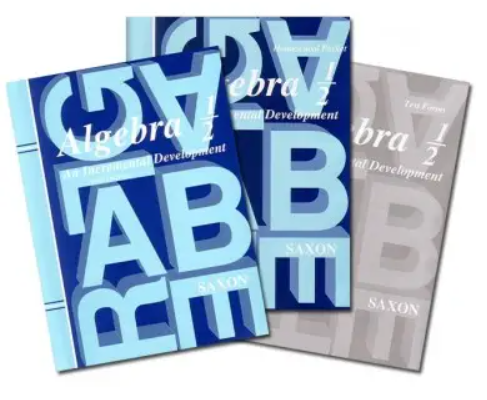Required Textbook: Saxon Algebra 1/2

Algebra 1/2 is made up of five instructional components: Introduction of the New Increment, Examples with Complete Solutions, Practice of the Increment, Daily Problem Set, and Cumulative Tests. Algebra 1/2 covers all topics normally taught in prealgebra, as well as additional topics from geometry and discrete mathematics. It is recommended for seventh-graders who plan to take first-year algebra in the eighth grade or for eighth-graders who plan to take first-year algebra in the ninth grade. Algebra 1/2 represents the culmination of the study of prealgebra mathematics. The textbook contains answers to odd-numbered problems, and an answer key is provided for all homework problems.

In Algebra 1/2, students will learn:

• fractions and their arithmetic operations
• decimals and their arithmetic operations
• mixed numbers and their arithmetic operations
• signed numbers and their arithmetic operations
• order of operations
• percents ratios
• proportions
• divisibility
• rounding
• place value
• unit conversions
• scientific notation
• evaluation and simplification of algebraic expressions
• the solution of linear equations in one unknown
• word problems involving algebraic concepts
• graphing
• perimeter area
• surface area
• volume
• classification of geometric figures and solids
• geometric construction
• symmetry
Live classes will meet weekly, 30 weeks, beginning the first week of September (see the posted Academic Schedule for holidays) on the same day and time each week, for a one-hour-live class. During class, the tutor will: explain the assignment for the coming week; answer your questions and perhaps pose some; note and address any problems or difficulties noticed in the prior week’s work; and lead a brief discussion of the topic at hand. These are rather informal and friendly live tutoring sessions.

Placement Information: Saxon books are skill-level books, not grade-level books. It is essential that each student is placed in the text that meets the skill level of the individual.Required Textbook: Saxon Algebra I

Algebra 1 is made up of five instructional components: Introduction of the New Increment, Examples with Complete Solutions, Practice of the Increment, Daily Problem Sets, and Cumulative Tests. Algebra 1 covers topics typically treated in a first-year algebra course.

Algebra 1 includes such topics as:

• arithmetic and evaluation of expressions involving signed numbers, exponents, and roots
• properties of real numbers absolute value
• equations and inequalities involving absolute value
• scientific notation
• unit conversions
• solution of equations in one unknown
• solution of simultaneous equations
• the algebra of polynomials and rational expressions
• word problems requiring algebra for the solution (such as uniform motion and coin problems)
• graphical solution of simultaneous equations
• graphs of a variety of functions: linear, quadratic, cubic, square root, absolute value, etc.
• translations and reflections of graphs factoring
• Pythagorean theorem
• algebraic proofs
• functional notation and functions
• solution of quadratic equations by factoring, completing the square, and quadratic formula
• direct and inverse variation
• exponential growth
• computation of the perimeter and area of two-dimensional regions
• computation of the surface area and volume of a wide variety of geometric solids
• statistics
• probability
Live classes will meet weekly, 30 weeks, beginning the first week of September (see the posted Academic Schedule for holidays) on the same day and time each week, for a one-hour-live class. During class, the tutor will: explain the assignment for the coming week; answer your questions and perhaps pose some; note and address any problems or difficulties noticed in the prior week’s work; and lead a brief discussion of the topic at hand. These are rather informal and friendly live tutoring sessions.

### Math 9th Grade – Algebra II

Placement Information: Saxon books are skill-level books, not grade-level books. It is essential that each student is placed in the text that meets the skill level of the individual.Required Textbook: Saxon Algebra II

Traditional second-year algebra topics, as well as a full semester of informal geometry, are included with both real-world, abstract and interdisciplinary applications. Topics include geometric functions like angles, perimeters, and proportional segments; negative exponents; quadratic equations; metric conversions; logarithms; and advanced factoring. Student Text is 558 pages, short answers for problem/practice sets, an index and glossary are included; hardcover. The Test book contains both student tests and solutions with work shown along with the final answer.

Why study mathematics? To form a well-ordered mind that can recognize some of the higher forms of beauty. Mathematics has always been seen as an essential part of the educational curriculum because of its uniquely humanizing effect in training the abstract, logical part of the mind.

This course utilizes the time-tested Saxon Math curriculum to cover all of the topics normally addressed in an Algebra II class. Algebra I is a prerequisite.

Live classes will meet weekly, 30 weeks, beginning the first week of September (see the posted Academic Schedule for holidays) on the same day and time each week,  for a one-hour-live class. During class, the tutor will: explain the assignment for the coming week; answer your questions and perhaps pose some; note and address any problems or difficulties noticed in the prior week’s work; and lead a brief discussion of the topic at hand. These are rather informal and friendly live tutoring sessions.

### Math 10th Grade – Geometry

Placement Information: Saxon books are skill-level books, not grade-level books. It is essential that each student is placed in the text that meets the skill level of the individual.

Required Textbook: Saxon GeometrySaxon Geometry is the perfect solution for students who prefer a dedicated geometry course…yet want Saxon’s proven methods! Presented in the familiar Saxon approach of incremental development and continual review, topics are continually kept fresh in students’ minds. Covering triangle congruence, postulates and theorems, surface area and volume, two-column proofs, vector addition, and slopes and equations of lines, Saxon features all the topics covered in a standard high school geometry course. Two-tone illustrations help students really “see” the geometric concepts, while sidebars provide additional notes, hints, and topics to think about. Parents will be able to easily help their students with the solutions manual, which includes step-by-step solutions to each problem in the student book; and quickly assess performance with the test book (test answers included). Tests are designed to be administered after every five lessons after the first ten.

Why study mathematics? To form a well-ordered mind that can recognize some of the higher forms of beauty. Throughout Western Civilization, great thinkers have found a particular beauty in Geometry. Mathematics has always been seen as an essential part of the educational curriculum because of its uniquely humanizing effect in training the abstract, logical part of the mind.

This course utilizes the time-tested Saxon Math curriculum to cover all of the topics normally addressed in a Geometry class. Algebra I is a prerequisite.

Live classes will meet weekly, 30 weeks, beginning the first week of September (see the posted Academic Schedule for holidays) on the same day and time each week,  for a one-hour-live class.  During class, the tutor will: explain the assignment for the coming week; answer your questions and perhaps pose some; note and address any problems or difficulties noticed in the prior week’s work; and lead a brief discussion of the topic at hand. These are rather informal and friendly live tutoring sessions.

Placement Information: Saxon books are skill-level books, not grade-level books. It is essential that each student is placed in the text that meets the skill level of the individual.Advanced Mathematics is made up of five instructional components: Introduction of the New Increment, Examples with Complete Solutions, Practice of the Increment, Daily Problem Sets, and Cumulative Tests. In Advanced Mathematics, topics from algebra, geometry, trigonometry, discrete mathematics, and mathematical analysis are interwoven to form a fully integrated text. A rigorous treatment of Euclidean geometry is also presented. Word problems are developed throughout the problem sets and become progressively more elaborate. With this practice, students will be able to solve challenging problems such as rate problems and work problems involving abstract quantities. The graphing calculator is used to graph functions and perform data analysis. Conceptually oriented problems that prepare students for college entrance exams (such as the ACT and SAT) are included in the problem sets.

Advanced Mathematics includes such topics as:

• permutations and combinations
• trigonometric identities
• inverse trigonometric functions
• conic sections
• graphs of sinusoids
• rectangular and polar representation of complex numbers
• De Moivre’s theorem
• matrices and determinants
• the binomial theorem
• the rational roots theorem
• Why study mathematics? To form a well-ordered mind that can recognize some of the higher forms of beauty. Mathematics has always been seen as an essential part of the educational curriculum
• because of its uniquely humanizing effect in training the abstract, logical part of the mind.

This course utilizes the time-tested Saxon Math curriculum to cover all of the topics normally addressed in a pre-calculus class. Algebra II and Geometry are prerequisites.

Live classes will meet weekly, 30 weeks, beginning the first week of September (see the posted Academic Schedule for holidays) on the same day and time each week, for a one-hour-live class. During class ,the tutor will: explain the assignment for the coming week; answer your questions and perhaps pose some; note and address any problems or difficulties noticed in the prior week’s work; and lead a brief discussion of the topic at hand. These are rather informal and friendly live tutoring sessions.

### Math 12th Grade – Calculus

Placement Information: Saxon books are skill-level books, not grade-level books. It is essential that each student is placed in the text that meets the skill level of the individual.

Required Textbook: Saxon Calculus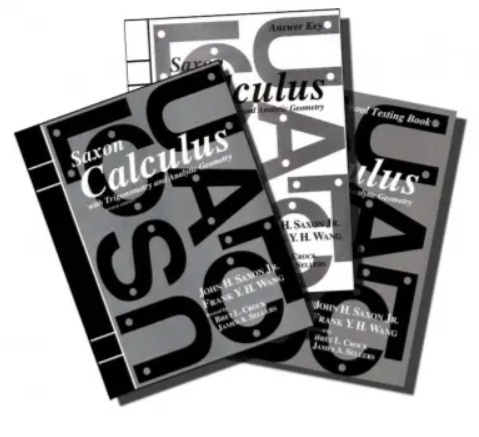Calculus is made up of four instructional components: Introduction of the New Increment, Examples with Complete Solutions, Daily Problem Sets, and Cumulative Tests. Calculus treats all the topics normally covered in an Advanced Placement AB-level calculus program, as well as many from a BC-level program. The text begins with a review of the mathematical concepts and skills required for calculus. In the early problem sets, students practice setting up word problems they will later encounter as calculus problems. The problem sets contain multiple-choice and conceptually-oriented problems similar to those found on the Advanced Placement examination. Whenever possible, an intuitive introduction precedes a rigorous examination of a concept. Proofs are provided for all important theorems. For example, three proofs, one intuitive and two rigorous, are given for the Fundamental Theorem of Calculus. Numerous applications to physics, chemistry, engineering, and business are also treated in both the lessons and the problem sets. Use of this text has allowed students to take the Advanced Placement examination and score well.

Why study mathematics? To form a well-ordered mind that can recognize some of the higher forms of beauty. Indeed, Calculus has a beauty all its own. Mathematics has always been seen as an essential part of the educational curriculum because of its uniquely humanizing effect in training the abstract, logical part of the mind.

This course utilizes the time-tested Saxon Math curriculum to cover all of the topics normally addressed in Calculus class. Advanced Mathematics, also known as Trigonometry or Pre-Calculus, is a prerequisite.

Live classes will meet weekly, 30 weeks, beginning the first week of September (see the posted Academic Schedule for holidays) on the same day and time each week, for a one-hour-live class. During class, the tutor will: explain the assignment for the coming week; answer your questions and perhaps pose some; note and address any problems or difficulties noticed in the prior week’s work; and lead a brief discussion of the topic at hand. These are rather informal and friendly live tutoring sessions.